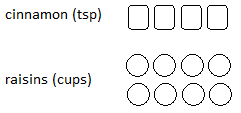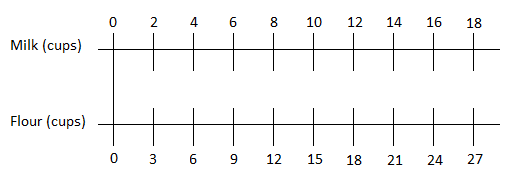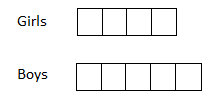# Understanding and Representing Ratios

Students are introduced to the concept of ratios, learning ratio language to describe the association between two or more quantities and different strategies to solve ratio problems.

## Unit Summary

In unit 1, sixth-grade students have the opportunity to study a concept that is brand new to them: ratios. They learn how to use ratio language to describe the association between two or more quantities, expanding their abilities to analyze relationships and see multiplicative patterns. Students learn many ways to represent ratios, starting with discrete drawings and working their way to abstract tables. These representations become important tools in their ratios toolkit, enabling students to be strategic about which tools to use for different problems (MP.5). When students work with tables and double number lines, they discover how structure can shine light on a relationship, especially when comparing multiple ratio situations (MP.7).

Throughout the unit, students see similar problems posed to them in different lessons. This is to support students learning new strategies to solve ratio problems and to compare and contrast different approaches. By the end of the unit, students should be able to select a strategy they think is best for a problem and to explain their choice.

In fourth and fifth grade, students learned the difference between multiplicative and additive comparisons and they interpreted multiplication as a way to scale. Students will access these prior concepts in this unit as they investigate patterns and structures in ratio tables and use multiplication to create equivalent ratios.

The work students do in this unit connects directly to Unit 2: Rates & Percent and re-appears in Unit 6: Equations and Inequalities when students analyze and graph relationships between independent and dependent variables. Beyond sixth grade, students extend their understanding of ratios and rates to investigate proportional relationships in seventh grade. This sets the groundwork for the study of functions, linear equations, and systems of equations, which students will study in eighth grade and high school.

This unit includes the MA-specific standard 6.RP.3e, which refers to a specific type of ratio problem about an object’s mass and volume. While this context is specific to this MA standard, it poses a real-world example of ratio problems that is worthwhile to solve.

Pacing: 21 instructional days (18 lessons, 2 flex days, 1 assessment day).

For guidance on adjusting the pacing for the 2021-2022 school year, see our 6th Grade Scope and Sequence Recommended Adjustments.• Unit Launch
• Expanded Assessment Package
• Problem Sets for Each Lesson
• Student Handout Editor
• Vocabulary Package

## Assessment

This assessment accompanies Unit 1 and should be given on the suggested assessment day or after completing the unit.

## Unit Prep

### Intellectual Prep

?

#### Internalization of Standards via the Post-Unit Assessment

• Take the Post-Unit Assessment. Annotate for:
• Standards that each question aligns to
• Strategies and representations used in daily lessons
• Relationship to Essential Understandings of unit
• Lesson(s) that Assessment points to

#### Internalization of Trajectory of Unit

• Read and annotate the Unit Summary.
• Notice the progression of concepts through the unit using the Lesson Map.
• Essential Understandings
• Connection to Post-Unit Assessment questions
• Identify key opportunities to engage students in academic discourse. Read through our Teacher Tool on Academic Discourse and refer back to it throughout the unit.

#### Unit-Specific Intellectual Prep

Model Example
Discrete drawing The ratio of teaspoons of cinnamon to cups of raisins is 4:8.Double number line For every 2 cups of milk, there are 3 cups of flour.Ratio table A turtle travels 3 feet every 9 seconds.
 Distance (ft) Time(sec) 3 9 6 18 9 27 30 90
Tape diagram The ratio of girls to boys in a 6th grade class is 4 to 5.### Essential Understandings

?

• A ratio is a set of numbers that associates two or more quantities. The order of the values in a ratio relates directly to the order of the quantities described.
• Equivalent ratios are useful in understanding a situation more deeply or in comparing multiple situations. Two ratios are equivalent if there is a nonzero number that can be multiplied by both quantities in one ratio to equal the corresponding quantities in the second ratio.
• Ratio problems can be represented and solved using a variety of strategies, tools, and representations, including a discrete diagram, a double number line, a ratio table, and tape diagrams. Each tool has its advantages and disadvantages and can be selected strategically.

### Vocabulary

?

double number line

tape diagram

ratio

part to part ratio

part to whole ratio

multiplicative relationship

equivalent ratio

ratio table

To see all the vocabulary for this course, view our 6th Grade Vocabulary Glossary.

### Materials

?

• Calculators (1 per student) — This lesson involves calculations with decimals. Students have worked with decimals in 5th grade, however, in order to keep the emphasis on the ratio reasoning, it is recommended to give students calculators to support their computations throughout this lesson (MP.5).
• Ratio Shapes Handout (1 per student)

# 1

6.RP.A.1

Define ratio and use ratio language to describe associations between two or more quantities.

# 2

6.RP.A.1

Represent ratios using discrete drawings. Understand that the order of numbers in a ratio matters.

# 3

6.RP.A.1

Define and find equivalent ratios.

# 4

6.RP.A.1

Reason with equivalent ratios and determine if two ratios are equivalent.

# 5

6.RP.A.3

Represent ratios using double number lines and identify equivalent ratios.

# 6

6.RP.A.3

Solve ratio problems using strategies including double number lines.

# 7

6.RP.A.3

6.RP.A.3.B

Find equivalent ratios using ratios with “per 1” unit.

# 8

6.RP.A.3

Compare situations using equivalent ratios and double number lines.

# 9

6.RP.A.3

Use ratio reasoning to solve a three-act task.

# 10

6.RP.A.3

6.RP.A.3.A

Represent ratios in tables.

# 11

6.RP.A.3

6.RP.A.3.A

Understand the structure of ratio tables. Solve ratio problems using tables.

# 12

6.RP.A.3

6.RP.A.3.A

Solve ratio problems using tables, including those involving total amounts.

# 13

6.RP.A.3.A

Compare ratios using tables.

# 14

6.RP.A.1

6.RP.A.3

6.RP.A.3.A

Solve ratio problems using different strategies.

# 15

6.RP.A.1

6.RP.A.3

Solve part:part ratio problems using tape diagrams.

# 16

6.RP.A.1

6.RP.A.3

Solve part:whole ratio problems using tape diagrams.

# 17

6.RP.A.3

Solve more complex ratio problems using tape diagrams.

# 18

6.RP.A.3

Solve ratio problems using a variety of strategies, including reasoning about diagrams, double number lines, tables, and tape diagrams. Summarize strategies for solving ratio problems.

## Common Core Standards

Key: Major Cluster Supporting Cluster Additional Cluster

### Core Standards

?

##### Ratios and Proportional Relationships
• 6.RP.A.1 — Understand the concept of a ratio and use ratio language to describe a ratio relationship between two quantities. For example, "The ratio of wings to beaks in the bird house at the zoo was 2:1, because for every 2 wings there was 1 beak." "For every vote candidate A received, candidate C received nearly three votes."

• 6.RP.A.3 — Use ratio and rate reasoning to solve real-world and mathematical problems, e.g., by reasoning about tables of equivalent ratios, tape diagrams, double number line diagrams, or equations.

• 6.RP.A.3.A — Make tables of equivalent ratios relating quantities with whole number measurements, find missing values in the tables, and plot the pairs of values on the coordinate plane. Use tables to compare ratios.

• 6.RP.A.3.B — Solve unit rate problems including those involving unit pricing and constant speed. For example, if it took 7 hours to mow 4 lawns, then at that rate, how many lawns could be mowed in 35 hours? At what rate were lawns being mowed?

?

• 4.MD.A.1

• 5.NF.B.3

• 5.NF.B.5

• 5.NF.B.5.A

• 5.NF.B.5.B

• 4.OA.A.2

• 5.OA.B.3

?

• 6.EE.C.9

• 6.RP.A.2

• 6.RP.A.3.C

• 6.RP.A.3.D

• 7.RP.A.1

• 7.RP.A.2

• 7.RP.A.3

### Standards for Mathematical Practice

• CCSS.MATH.PRACTICE.MP1 — Make sense of problems and persevere in solving them.

• CCSS.MATH.PRACTICE.MP2 — Reason abstractly and quantitatively.

• CCSS.MATH.PRACTICE.MP3 — Construct viable arguments and critique the reasoning of others.

• CCSS.MATH.PRACTICE.MP4 — Model with mathematics.

• CCSS.MATH.PRACTICE.MP5 — Use appropriate tools strategically.

• CCSS.MATH.PRACTICE.MP6 — Attend to precision.

• CCSS.MATH.PRACTICE.MP7 — Look for and make use of structure.

• CCSS.MATH.PRACTICE.MP8 — Look for and express regularity in repeated reasoning.